Check the below Online Education NCERT MCQ Questions for Class 7 Maths Chapter 6 The Triangle and its Properties with Answers Pdf free download. MCQ Questions for Class 7 Maths with Answers were prepared based on the latest exam pattern. We have provided The Triangle and its Properties Class 7 Maths MCQs Questions with Answers to help students understand the concept very well. https://ncertmcq.com/mcq-questions-for-class-7-maths-with-answers/

Students can also refer to NCERT Solutions for Class 7 Maths Chapter 6 The Triangles and its Properties for better exam preparation and score more marks.

## Online Education for The Triangle and its Properties Class 7 MCQs Questions with Answers

Class 7 Maths Chapter 6 MCQ Question 1.
How many elements are there in a triangle?
(a) 3
(b) 6
(c) 4
(d) None of these.

Hint:
See a triangle.

Triangle And Its Properties Class 7 MCQ Question 2.
How many vertices does a triangle have?
(a) 1
(b) 2
(c) 3
(d) 4

Hint:
See a triangle.

Class 7 Maths Chapter 6 MCQ With Answers Question 3.
How many sides are there in a triangle?
(a) 1
(b) 2
(c) 3
(d) 4

Hint:
See a triangle.

MCQ Questions For Class 7 Maths Chapter 6 Question 4.
How many angles are there in a triangle?
(a) 1
(b) 2
(c) 3
(d) 4

Hint:
See a triangle.

Class 7 Maths Ch 6 MCQ Question 5.
If two sides of a triangle are not equal, the triangle is called
(a) scalene
(b) isosceles
(c) equilateral
(d) right-angled

Hint:
Definition of a scalene triangle.

Class 7 Math Chapter 6 MCQ Question 6.
If two sides of a triangle are equal, the triangle is called
(a) isosceles
(b) equilateral
(c) scalene
(d) right-angled

Hint:
Definition of an isosceles triangle.

MCQ On Triangle And Its Properties Class 7 Question 7.
If all the three sides of a triangle are equal, the triangle is called
(a) equilateral
(b) right-angled
(c) isosceles
(d) scalene

Hint:
Definition of an equilateral triangle.

Class 7 Chapter 6 Maths MCQ Question 8.
If all the angles of a triangle are acute, the triangle is called
(a) obtuse-angled
(b) acute-angled
(c) right-angled
(d) none of these

Hint:
Definition of an acute-angled triangle.

MCQ On Triangles Class 7 Question 9.
If one angle of a triangle measures 90°, the triangle is called
(a) acute-angled
(b) obtuse-angled
(c) right-angled
(d) none of these

Hint:
Definition of a right triangle.

Triangles And Its Properties Class 7 MCQ Question 10.
If one angle of a triangle is obtuse, the triangle is called
(a) acute-angled
(b) obtuse-angled
(c) right-angled
(d) none of these

Hint:
Definition of an obtuse angled triangle.

Ncert Class 7 Maths Chapter 6 MCQ Question 11.
How many medians can a triangle have?
(a) 1
(b) 2
(c) 3
(d) 4

Hint:
Draw medians and count.

Class 7th Maths Chapter 6 MCQ Question 12.
How many altitudes can a triangle have?
(a) 1
(b) 2
(c) 3
(d) 4

Hint:
Draw altitudes and count.

MCQ Questions For Class 7 Maths Triangle And Its Properties Question 13.
The total measure of the three angles of a triangle is
(a) 360°
(b) 90°
(c) 180°
(d) none of these

Hint:
Angle Sum Property of a triangle.

Class 7 Triangle And Its Properties MCQ Question 14.
The measure of each angle of an equilateral triangle is
(a) 30°
(b) 45°
(c) 90°
(d) 60°

Hint:
x° + x° + x° = 180° ⇒ x° = 60°.

MCQ Class 7 Maths Chapter 6 Question 15.
Which of the following statements is true?
(a) A triangle can have two right angles
(b) A triangle can have two obtuse angles
(c) A triangle can have two acute angles
(d) A triangle can have all the three angles less than 60°

Answer: (c) A triangle can have two acute angles

Question 16.
Which of the following statements is true?
(a) A triangle can have all the three angles equal to 60°.
(b) A triangle can have all the three angles greater than 60°.
(c) The sum of any two angles of a triangle is always greater than the third angle.
(d) The difference between the lengths of any two sides of a triangle is greater than the length of the third side

Answer: (a) A triangle can have all the three angles equal to 60°.

Question 17.
Which of the following statement is false?
(a) The sum of the lengths of any two sides of a triangle is less than the third side.
(b) In a right-angled triangle, the square on the hypotenuse = sum of the squares on the legs.
(c) If the Pythagorean property holds, the triangle must be right-angled.
(d) The diagonal of a rectangle produce ‘by itself the same area as produced by its length and breadth

Answer: (a) The sum of the lengths of any two sides of a triangle is less than the third side.

Question 18.
Two angles of a triangle measure 90° and 30°. The measure of the third angle is
(a) 90°
(b) 30°
(c) 60°
(d) 120°

Hint:
Third angle = 180° – (90° + 30°) = 60°.

Question 19.
The ratio of the measures of the three angles of a triangle is 2 : 3 : 4. The measure of the largest angle is
(a) 80°
(b) 60°
(c) 40°
(d) 180°

Hint:
Largest angle = $$\frac { 4 }{ 2+3+4 }$$ × 180° = 80°.

Question 20.
In the following figure, the side BC of ∆ ABC is extended up to the point D. If ∠A = 55° and ∠B = 60°, then the measure of ∠ACD is(a) 120°
(b) 110°
(c) 115°
(d) 125°

Hint:
∠ACD = 60° + 55° = 115°.

Question 21.
In the following figure, the measure of ∠A is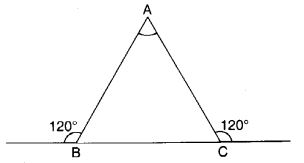(a) 30°
(b) 45°
(c) 90°
(d) 30°

Hint:
∠A – 180° – [(180° – 120°) + (180° – 120°)] = 60°.

Question 22.
In the following figure, the measure of ∠A is(a) 70°
(b) 90°
(c) 80°
(d) 100°

Hint:
∠A – 180° – (60° + 40°) = 80°

Question 23.
In the following figure, m || QR. Then, the measure of ∠QPR is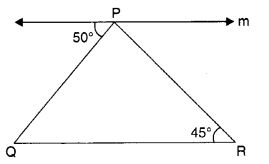(a) 80°
(b) 85°
(c) 75°
(d) 70°

Hint:
∠PQR = 50°
∴ ∠QPR – 180° – (50° + 45°) = 85°.

Question 24.
In the following figure, find ∠ x and ∠ y, if ∠x – ∠y – 10°(a) 65°, 55°
(b) 55°, 45°
(c) 45°, 35°
(d) 60°, 60°

Answer: (a) 65°, 55°
Hint:
∠x + ∠y = 120°; ∠x – ∠y = 10° Solve to get ∠x = 65°, ∠y = 55°.

Question 25.
In the following figure, find ∠ B.(a) 30°
(b) 45°
(c) 40°
(d) 60°

Hint:
∠B = 180° – [(180° – 110°) + 50°] = 60°.

Question 26.
In the following figure, ∆ ABC is an equilateral triangle. Find ∠x.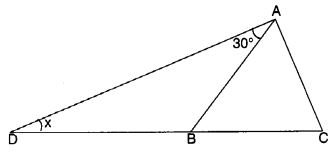(a) 30°
(b) 45°
(c) 60°
(d) 90°

Hint:
∠ABC = 60°.
∴ ∠ABD = 180° – 60° = 120°
∴ x = 180° – (120° + 30°) = 30°.

Question 27.
In the following figure, one angle of triangle ABC is 40°. If the difference of the other two angles is 30°, find the larger of the other two angles.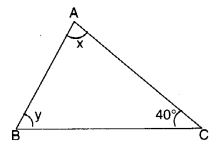(a) 85°
(b) 80°
(c) 75°
(d) 70°

Hint:
x + y = 180° – 40° = 140° x – y = 30°. Solve to get x = 85°.

Question 28.
In the following figure, find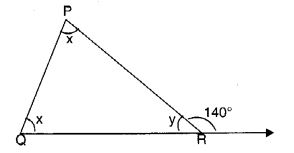(a) 60°
(b) 70°
(c) 80°
(d) 75°

Hint:
x + x = 140° ⇒ x = 70°.

Question 29.
In the following figure, find x if BA || CE.(a) 60°
(b) 40°
(c) 45°
(d) 65°

Hint:
∠ECD = ∠ABC = 50°;
∴ x = 180° – (65° + 50°) = 65°.

Question 30.
Find the value of the unknown interior angle x in the following figure:(a) 30°
(b) 35°
(c) 40°
(d) 45°

Hint:
x + 90° = 130° ⇒ x = 40°.

Question 31.
Find the value of unknown x in the following figure: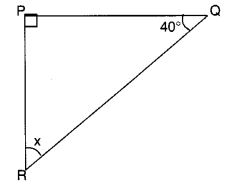(a) 40°
(b) 50°
(c) 45°
(d) 55°

Hint:
x + 40° + 90° = 180° ⇒ x = 50°.

Question 32.
Find the value of unknown x in the following figure:(a) 10°
(b) 15°
(c) 20°
(d) 25°

Hint:
x + 5x + 90° = 180° ⇒ x = 15°.

Question 33.
Find angle x in the following figure: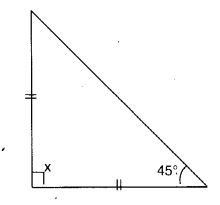(a) 90°
(b) 80°
(c) 95°
(d) 100°

Hint:
x + 45 + 45° = 180° ⇒ x = 90°.

Question 34.
Find angle x in the following figure: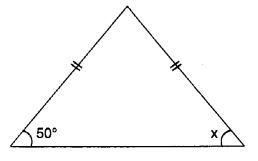(a) 40°
(b) 50°
(c) 45°
(d) 60°

Hint:
x = 50°.

Question 35.
Find angle x in the following figure: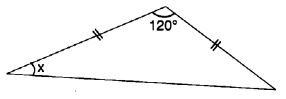(a) 40°
(b) 30°
(c) 25°
(d) 35°

Hint:
x + x + 120° = 180° ⇒ x = 30°.

Question 36.
Find angle x in the following figure:(a) 40°
(b) 45°
(c) 35°
(d) 50°

Hint:
x + 140° = 180° ⇒ x = 40°.

Question 37.
Find angle x in the following figure: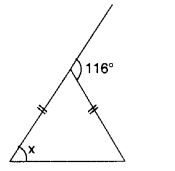(a) 58°
(b) 59°
(c) 57°
(d) 56°

Hint:
x + x = 116° ⇒ x = 58°.

Question 38.
Find angle x in the following figure: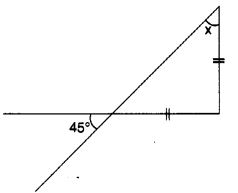(a) 45°
(b) 40°
(c) 35°
(d) 50°

Hint:
x = 45°.

Question 39.
In which case of the following lengths of sides of a triangle, is it possible to draw a triangle?
(а) 3 cm, 4 cm, 7 cm
(b) 2 cm, 3 cm, 7 cm
(c) 3 cm, 4 cm, 5 cm
(d) 3 cm, 3 cm, 7 cm

Answer: (c) 3 cm, 4 cm, 5 cm
Hint:
3 + 4 > 5; 4 + 5 > 3; 5 + 3 > 4.

Question 40.
Which of the following cannot be the sides of a right triangle?
(а) 2 cm, 2 cm, 4 cm
(b) 5 cm, 12 cm, 13 cm
(c) 6 cm, 8 cm, 10 cm
(d) 3 cm, 4 cm, 5 cm